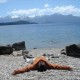## Tutorial on using the rworldmap package

This blog following up my previous oneattempts to explain how the geo-pie map was created.

I do not know how to attach a .rflow file in this blog. What you can do is to copy the following code into Notepad and save it as XXX.rflow, and open it by RAnalyticFlow. By using it, you can see the task is divided into 7 steps, and run up to any node you want.

Before you run the code below, you have make sure that required packages are installed. If you would like to run the 7th part of the code, you need to download an image of R-bloggers. I hope to make a generalized function out the code ONE DAY, but without any promises though. After running the code, you will haveHere is the plain R code.

```################# 1. Clear working space ###############
rm(list=ls())

## make sure you have them installed
require(doBy)
require(plyr)
require(rworldmap)
require(TeachingDemos)

################ 3. make trade data #################
### make sure your country names is by UN convention
### Otherwise you need to modify your countries names
"United States",
"United Kingdom",
"Brazil",
"Indonesia",
"Germany",
"Russia",
"Australia",
"Malaysia",
"South Africa")
)

### fabricate some data for each country
### I just generate numbers ramdomly and make sure
### they are positive

################## 4. make our Geo-pie plots #################
### "?mapPies" commands without the quotes

##### 4.1 merging with existing data
joinCode = "NAME",
nameJoinColumn = "Country")

## This the data that we will plot
dF <- sPDF@data

### make our pie plot
par(mai= c(0,0,0.6,0),
xaxs = "i",
yaxs = "i")

mapPies(dF =dF,
nameX="LON",
nameY="LAT",
nameZs =c("product1",
"product2",
"product3",
"product4",
"product5") ,
zColours=c("red",
"green",
"magenta",
"yellow",
"blue"),
oceanCol = "lightblue",
landCol = "wheat",
mapRegion="world",
xlim=c(-181,181),
ylim=c(-81,80))

title(main=paste("Our lovely Geo-Pie plot using rworldmap package"),
cex=3)

legend(-180.1516,90,
legend=c("Product 1",
"Product 2",
"Product 3",
"Product 4",
"Product 5"),
col=c("red",
"yellow",
"green",
"magenta",
"blue"),
pch=16,
cex=0.8,
pt.cex=1.5,
bty="o",
box.lty=0,
horiz = F,
bg="#FFFFFF70")

################ 5. Lets make a barplot on our map ##############
## derive the data for barplot -- top 10 markets for
## product1
tmp_product1 <- orderBy(~-product1,dF)[1:10,]

### make the barplot as a function
subp1.1 <- function(...){
barplot(rev(tmp_product1\$product1),
width=1,
names.arg=rev(tmp_product1\$Country),
las=2,
border="transparent",
horiz=T,
col="darkgrey",
main=paste("Top 10 markets for product 1"),
cex.main=0.7
)
mtext(1,
text="NZ\$ million",
line=1.7,
cex=0.7
)
grid(col="black")
}

################# 6. plot the barplot and make a bit more lovely ##
#### make our background for the barplot
#### you have to fiddle a bit on the position
subplot(rect(-180,-90,-100,-32,
col="#FFFFFF70",
border=NA),
x=0,
y=0,

#### let's plot
w_x <- 0.66
w_y <- 1
pos <- c(-160,-81)

par(mar=c(4,4,1,1),
cex.axis=0.7,
cex.lab=0.7,
bg="white",
cex.main=0.7)

subplot(subp1.1(),
x=pos,
y=pos,
size=c(w_x,w_y)
)
############ 7. (Optional) put a logo on your map ###############
#Get the plot information so the image will fill the plot box, and draw it
pos_rblog <- c(150.7,58.4,181,73.8)
rasterImage(rblog,
pos_rblog,
pos_rblog,
pos_rblog,
pos_rblog)
rect(pos_rblog,
pos_rblog,
pos_rblog,
pos_rblog,border="black",
)```1.Hugh says:
2.Irene says: